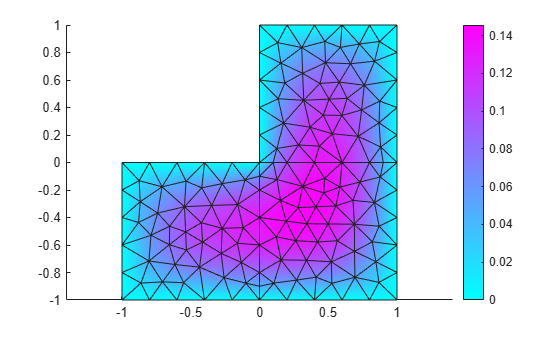# tri2grid

(Not recommended) Interpolate from PDE triangular mesh to rectangular grid

`tri2grid` is not recommended. Use `interpolateSolution` instead. For more information, see Compatibility Considerations.

## Syntax

``uxy = tri2grid(p,t,u,x,y)``
``[uxy,tn,a2,a3] = tri2grid(p,t,u,x,y)``
``uxy = tri2grid(p,t,u,tn,a2,a3)``

## Description

example

````uxy = tri2grid(p,t,u,x,y)` computes the interpolated function values `uxy` over the grid defined by the vectors `x` and `y` using the function values `u` on the triangular mesh defined by `p` and `t`.`tri2grid` uses linear interpolation in the triangle containing the grid point.```

example

````[uxy,tn,a2,a3] = tri2grid(p,t,u,x,y)` also returns the index `tn` of the triangle containing each grid point, and interpolation coefficients `a2` and `a3`.```
````uxy = tri2grid(p,t,u,tn,a2,a3)` uses the values `tn`, `a2`, and `a3` returned by prior `tri2grid` call using the previous syntax to interpolate `u` to the same grid as in the prior call. This syntax is efficient for interpolating several functions to the same grid, such as interpolating hyperbolic or parabolic solutions at multiple times.```

## Examples

collapse all

Generate a triangular mesh of the L-shaped membrane. The geometry of the L-shaped membrane is described in the file `lshapeg`.

`[p,e,t] = initmesh('lshapeg');`

Solve an elliptic PDE on the L-shaped geometry.

`u = assempde('lshapeb',p,e,t,1,0,1);`

Plot the solution and the mesh.

`pdeplot(p,e,t,'XYData',u,'Mesh','on')`Interpolate the solution to the rectangular grid defined by the vectors `x` and `y`.

```x = -1:0.1:1; y = x; uxy = tri2grid(p,t,u,x,y);```

Plot the interpolated solution and the rectangular grid.

`surface(x,y,uxy)`For comparison, plot the original solution and the rectangular grid.

```[~,tn,a2,a3] = tri2grid(p,t,u,x,y); pdeplot(p,e,t,'XYGrid','on','GridParam',[tn;a2;a3], ... 'XYData',u,'Mesh','on')```## Input Arguments

collapse all

Mesh points, specified as a 2-by-`Np` matrix of points (nodes), where `Np` is the number of nodes in the mesh. For details on mesh data representation, see `initmesh`.

Data Types: `double`

Mesh elements, specified as a `4`-by-`Nt` matrix of triangles, where `Nt` is the number of triangles in the mesh. For details on mesh data representation, see `initmesh`.

Data Types: `double`

PDE solution, specified as a vector. For systems of equations, `tri2grid` interpolates only the first component. For solutions returned by `hyperbolic` or `parabolic`, pass `u` as a vector of values at one time, `u(:,k)`.

x-coordinates for rectangular grid, specified as a vector of elements in ascending order.

Data Types: `double`

y-coordinates for rectangular grid, specified as a vector of elements in ascending order.

Data Types: `double`

## Output Arguments

collapse all

Interpolated values, returned as an `ny`-by-`nx` matrix, where `nx` and `ny` are the lengths of the vectors `x` and `y`, respectively.

`tri2grid` uses linear interpolation in the triangle containing the grid point. At grid points outside of the triangular mesh, interpolated values are `NaN`.

Indices of triangles containing each grid point, returned as an `ny`-by-`nx` matrix, where `nx` and `ny` are the lengths of the vectors `x` and `y`, respectively. At grid points outside of the triangular mesh, indices of triangles are `NaN`.

Interpolation coefficient, returned as an `ny`-by-`nx` matrix, where `nx` and `ny` are the lengths of the vectors `x` and `y`, respectively. At grid points outside of the triangular mesh, values of the interpolation coefficients are `NaN`.

Interpolation coefficient, returned as an `ny`-by-`nx` matrix, where `nx` and `ny` are the lengths of the vectors `x` and `y`, respectively. At grid points outside of the triangular mesh, values of the interpolation coefficients are `NaN`.

## Version History

Introduced before R2006a

expand all